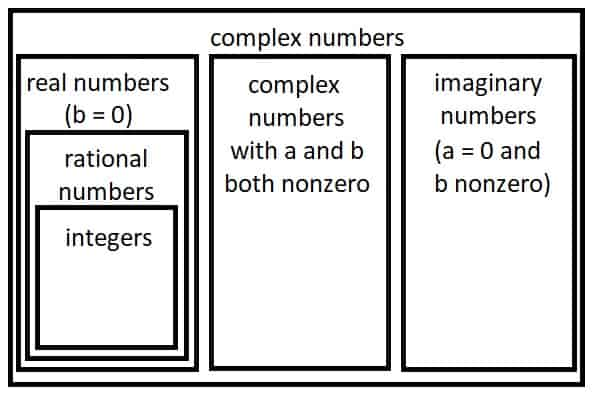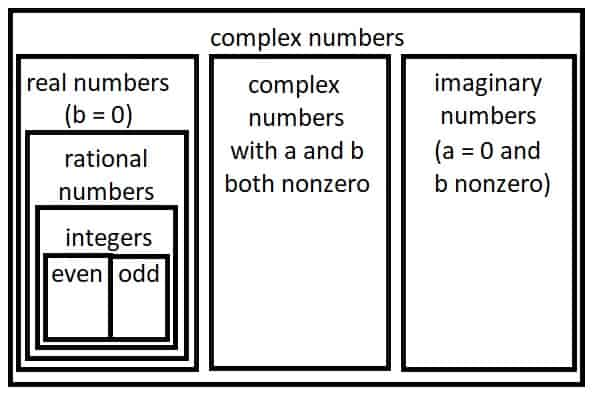# Complex Numbers (7 Common Questions Answered)

Complex numbers come up in algebra, calculus, and other areas of mathematics.  This set of numbers often raises some questions about its nature in relation to other sets.

Complex numbers contain the set of real numbers, rational numbers, and integers.  So, some complex numbers are real, rational, or integers.  The conjugate of a complex number is often used to simplify fractions or factor polynomials that are irreducible in the real numbers.  The modulus of a complex number gives us information about where a complex number lies in the coordinate plane.

Of course, complex numbers cannot be ordered like real numbers, since they are not on a line, but rather in an entire plane.  This is because the imaginary part adds a 2nd dimension when we graph complex numbers.

Let’s get started.

## Can A Complex Number Be A Real Number?

A complex number can be a real number in some cases, when its imaginary part is zero (b = 0). The set of real numbers is a subset of the set of complex numbers.

Remember that a complex number has the form

a + bi

where a and be are real numbers (a is the real part and b is the imaginary part of the complex number).

There are two special cases for complex numbers:

• Pure Imaginary Numbers (a = 0): this gives us a number of the form 0 + bi or just bi, which is a pure imaginary number for any nonzero b.  Some examples are i (a = 0, b = 1) and -2i (a = 0, b = -2).
• Real Numbers (b = 0): this gives us a number of the form a + 0i or just a, which is a real number for any a.  Some examples are 7 (a = 7, b = 0) and -5 (a = -5, b = 0).

From the 2nd special case, we can see that a complex number is a real number if its imaginary part is zero (that is, b = 0).  These numbers have the form a + 0i.

Alternatively, we can write any real number a as a complex number by writing it as a + 0i.

The diagram below illustrates the relationship between complex, real, and pure imaginary numbers.

## Can A Complex Number Be Rational?

A complex number can be rational if its complex part is zero (b = 0) and its real part is rational (a is rational).  This is due to the fact that the set of rational numbers is a subset of the set of real numbers.

One example is the rational number ½, which can be written as ½ + 0i (a = ½, b = 0).

Another example is the rational number -13/2, which can be written as -13/2 + 0i (a = -13/2, b = 0).

Note that a complex number can have both a and b rational, but these complex numbers are not rational numbers if b is nonzero.

For example, ½ + (2/3)i has a = ½ and b = 2/3, which are both rational.  However, the complex number ½ + (2/3)i itself is not rational.

The number (4/5)i has a = 0 and b = 4/5, which are both rational.  However, (4/5)i is not a rational number.

The diagram below adds rational numbers to the diagram showing the relationship between complex, real, and pure imaginary numbers.

## Can A Complex Number Be An Integer?

A complex number can be an integer if its complex part is zero (b = 0) and its real part is an integer (a is an integer).  This is due to the fact that the set of integers is a subset of the set of rational numbers (which is a subset of the set of real numbers).

One example is the integer 3, which can be written as 3 + 0i (a = 3, b = 0).

Another example is the integer -8, which can be written as -8 + 0i (a = -8, b = 0).

Note that a complex number can have both a and b as integers, but these complex numbers are not integers if b is nonzero.

For example, 2 + 5i has a = 2 and b = 5, which are both integers.  However, the complex number 2 + 5i itself is not an integer.

The number 6i has a = 0 and b = 6, which are both integers.  However, 6i is also not an integer.

The diagram below adds integers to the diagram showing the relationship between complex, real, and pure imaginary numbers.This diagram shows the relationship between complex, real, and imaginary numbers, along with rational numbers and integers.

## Can A Complex Number Be Even Or Odd?

A complex number can be even if its real part is an even integer and its imaginary part is zero (a = 2n for some integer n and b = 0).

A complex number can be odd if its real part is an odd integer and its imaginary part is zero (a = 2n + 1 for some integer n and b = 0).

Any complex number with nonzero b cannot be even or odd (since it is not an integer).This diagram shows the relationship between complex, real, and imaginary numbers, along with rational numbers, and integers (both even and odd).

## What Is The Conjugate Of A Complex Number?

For a complex number a + bi, its conjugate (or complex conjugate) is a – bi.

In other words, find the conjugate of a complex number, keep the real part the same, but take the opposite (negative) of the imaginary part.

For example, the conjugate of 2 + 3i is 2 – 3i.

The conjugate of 8 – 6i is 8 + 6i.

The conjugate of -4 – i is -4 + i.

The conjugate of 7i is -7i (The conjugate of any pure imaginary number is the opposite (negative) of the number.)

The conjugate of 5 is 5 (The conjugate of any real number is the number itself.)

[table of sample complex numbers and conjugates]

The product of any complex number and its conjugate is a real number, since:

• (a + bi)(a – bi)  [product of a complex number and its conjugate]
• a2 – abi + abi – b2i2  [FOIL]
• a2 – b2i2  [combine like terms]
• a2 + b2  [since i2 = -1, by definition of the imaginary unit i]

Since a and b are simply real numbers, then so are a2 and b2, and so is their sum, a2 + b2.

The key is that the imaginary parts cancel each other out when we combine like terms after we FOIL.

Complex conjugates are often used to convert the denominator of a complex fraction to a real number.

For example, if we have 1 / 2i, we can multiply by the complex conjugate -2i on the top and bottom of the fraction to get:

• 1 / 2i  [original fraction]
• (1*-2i) / (2i*-2i) [multiply by -2i on the top and bottom]
• -2i / -4i2
• -2i / 4  [since i2 = -1, by definition of the imaginary unit i]
• -i / 2

This fraction has a real number in the denominator.

We can also use complex conjugates to factor polynomials that are irreducible over the real numbers.

For example, f(x) = x2 + 1 is irreducible in the real numbers.

However, we can factor it as f(x) = (x + i)(x – i) in the complex numbers.

Note that i and –i are complex conjugates.

## What Is The Modulus Of A Complex Number?

The modulus of a complex number is the square root of the sum of the squares of its real and imaginary parts.  So, for a complex number z = a + bi, the modulus is |z| = (a2 + b2)1/2 which is a nonnegative real number.

Due to the notation |z|, which denotes the modulus of z, we also use the phrase “absolute value of z”, since |z| tells us the distance from z to the origin in the complex plane.

For example, the complex number z = 3 + 4i has a modulus of |z| = (32 + 42)1/2 = (9 + 16)1/2 = (25)1/2 = 5. This means that if we graph z = 3 + 4i in the complex plane, it is a distance of 5 units from the origin (the point 0 + 0i).

Note that the modulus of a complex number is the same as the square root of the product of the complex number and its conjugate.  So, for the complex number z = a + bi:

• (a + bi)(a – bi)  [product of a + bi and its complex conjugate]
• a2 – abi + abi – b2i2  [FOIL]
• a2 – b2i2  [combine like terms]
• a2 + b2  [since i2 = -1, by definition of the imaginary unit i]
• (a2 + b2)1/2 [take the square root]
• |z|  [by definition of the modulus of z = a + bi]

The modulus of a complex number is similar to absolute value in that it is never negative, and it is only zero for the complex number 0 + 0i (a = 0 and b = 0).

## Can A Complex Number Be Negative?

A complex number cannot be negative – this idea of negative or positive does not make sense for complex numbers.

A real number can be negative, since we can compare its position to zero on the number line:

• If a real number is to the left of zero on the number line, it is negative.
• If a real number is to the right of zero on the number line, it is positive.

However, we cannot graph complex numbers on a line as we can the real numbers.  This is because there is an extra dimension: the imaginary part of the complex number, or bi.

As a result, we must graph a complex number on a plane, rather than on a line.  As such, the concept of “left or right of zero” loses its meaning in two dimensions.

So, there is no concept of “negative” or “positive” complex numbers as there is for the real numbers.  Another way of saying this is that we cannot order the complex numbers in the way we can order the real numbers.

With that being said, we can still have complex numbers with a negative real part (a < 0), a negative imaginary part (b < 0), or both (a < 0 and b < 0).  However, we could not say that a + bi is negative in any of these cases.

We can also take the negative (opposite) of any complex number by changing the sign of both a and b.  For example, the negative (opposite) of 2 – 3i is -2 + 3i.

## Conclusion

Now you know more about complex numbers and how they relate to other number sets.  You also know about the conjugate, argument, and modulus of a complex number and what they can tell us.